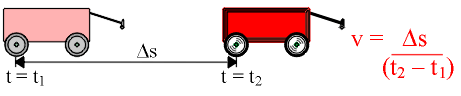### The measurement of wave speed, showing different factors that influence it.

WATCH THE VIDEOS

Two different media

Two frequencies

Theory:

The speed, v, of a moving object traveling between two points is the distance between those two points (Ds) divided by the time it takes to travel that distance (Dt = t2 - t1)A traveling wave is different from a moving object in that a wave is just a disturbance in a medium; no material moves in the direction of propagation of a wave. Still, if we pick a feature of a traveling wave - a certain crest on the wave, for example - we can measure its speed and call that the speed of the wave.

The speed of a wave does not depend on the amplitude of the wave. This can be shown by sending one large and one small wave through the same medium. They will both travel at the same speed.

The speed of a wave does depend on the medium through which the wave travels. This can be shown by creating waves in two different media (e.g. the large and the small wave machines). Waves in one medium travel more quickly than they do in the other.

Apparatus

• small and large wave machines
• stopwatch
• measuring stick

Procedure:

Measuring the Speed of a Wave:

• Set up the large wave machine on a flat surface.
• To create a single wave traveling down the wave demonstrator, lift the nearest rod up a few centimeters, and quickly flick the rod back to its original position. You will see a small wave travel down the rods to the end of the machine and reflect back again.
• Use a stopwatch to time how long it takes the wave to complete one length of the apparatus.
• With the time now recorded, measure the length of the apparatus, and you can now calculate the velocity at which the wave was traveling by using the equation velocity = distance / time. The wave in the videos travels about 88 cm in a time of 1.99 s, so the wave's velocity is v = (88 cm)/(1.99 s) = 44 cm/s = 0.44 m/s.

Amplitude:

• To show that the speed of a wave doesn't depend on the amplitude of the wave, set up the large wave machine as before.
• One after another, create two traveling waves of different amplitude. The distance between them will not change as they travel down the wave machine, so their speeds are the same.

Waves in Two Different Media:

• Set up the large wave machine next to the small wave machine.
• Simultaneously create identical waves in each wave machine. You will see that the wave in the large demonstrator takes much longer to reach the end of the apparatus than the wave in the small one does.